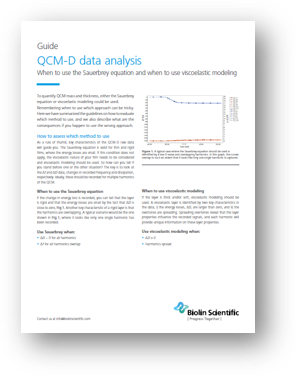# The Sauerbrey equation or viscoelastic modeling

- when you should use what, and what the consequences are if you use the wrong approach

To quantify QCM mass and thickness, either the Sauerbrey equation or viscoelastic modeling could be used. Here we have summarized the guidelines on how to evaluate which method to use, and we also describe what are the consequences if you happen to use the wrong approach.

In this guide you will find:• Check lists to evaluate which analysis method to use
• Data examples of the two situations
• An explanation of the consequences if the wrong data analysis approach is used